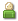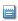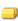# Electron Configuration For Nitrogen: Demystifying The Electronic Setup Of Nitrogen AtomJan 20th, 2023UncategorizedYou may have heard about the concept of electron configuration in chemistry, but it can be a daunting topic to understand. However, it is an essential concept to comprehend to have a better understanding of the behavior of atoms and molecules. In this article, we will demystify the electronic setup of nitrogen atom, one of the most crucial elements in the periodic table, and explain its electron configuration.

Nitrogen is the seventh element in the periodic table and has an atomic number of 7. Its electron configuration is crucial in determining its chemical properties and how it reacts with other elements. Understanding its electronic setup can help you better understand how it behaves in different chemical reactions and why it is an essential element for life on Earth. So let’s dive into the electron configuration of nitrogen and unravel the mystery behind this fascinating element.

## Understanding Electron Configuration

Let’s dive into how nitrogen’s electrons are arranged and get ready to be amazed by the science behind it all. Understanding electron configuration is crucial in comprehending the chemical and physical properties of elements. It refers to the distribution of electrons in an atom’s various energy levels or orbitals. Each orbital has a specific amount of electrons that it can hold, and the electrons in an atom’s outermost shell, or valence shell, are responsible for chemical bonding.

Electron spin is a fundamental property of electrons that distinguishes them from other subatomic particles. Electrons spin in one of two directions, either clockwise or counterclockwise, and they are represented by arrows pointing up or down. The Pauli exclusion principle states that no two electrons in an atom can have the same set of four quantum numbers, including their spin. Therefore, each orbital can only hold a maximum of two electrons with opposite spins.

## The Electronic Setup of Nitrogen Atom

To understand the electronic setup of nitrogen, it is important to first understand the number of electrons in the atom. Nitrogen has a total of 7 electrons, with 2 in the first energy level and 5 in the second energy level. The specific arrangement of electrons in nitrogen follows the Aufbau principle, where electrons fill the lowest energy levels first before moving to higher energy levels.

### The Number of Electrons in Nitrogen

You may be wondering how many particles make up the nitrogen element. Nitrogen has a total of 7 electrons, which are negatively charged particles that orbit around the nucleus of an atom. The electrons in nitrogen are arranged in shells or energy levels, with the first shell containing a maximum of 2 electrons, and the second shell holding a maximum of 8 electrons. Since nitrogen only has 7 electrons, it means that the first shell is fully occupied, while the second shell has only 5 electrons.

Valence electrons are the electrons in the outermost shell of an atom that are involved in chemical reactions. In nitrogen, the valence electrons are the electrons in the second shell, specifically the 2p orbitals. The orbital filling order for nitrogen is 1s2, 2s2, 2p3, which means that the first shell is filled with 2 electrons, the second shell has 2 electrons in the 2s orbital and 3 electrons in the 2p orbitals. Understanding the number of electrons and the valence electrons in nitrogen is crucial to understanding how nitrogen behaves in chemical reactions. In the subsequent section, we will delve into the specific arrangement of electrons in nitrogen.

### The Specific Arrangement of Electrons in Nitrogen

Understanding the arrangement of electrons in nitrogen is essential in comprehending how the element interacts in various chemical reactions. Nitrogen has a total of seven electrons, with two electrons in the first energy level and five electrons in the second energy level. The orbital distribution of these electrons follows the quantum mechanics principles, where each energy level can only accommodate a specific number of electrons.

In the second energy level of nitrogen, there are three sub-levels, namely s, p, and d. The s sub-level can hold a maximum of two electrons, while the p sub-level can hold up to six electrons. In nitrogen, the three p sub-level electrons are distributed in three separate orbitals, with each orbital containing one electron. This arrangement is referred to as a half-filled p sub-level, and it is responsible for the unique properties of nitrogen, such as its high reactivity and ability to form multiple bonds. In summary, the specific arrangement of electrons in nitrogen follows the principles of quantum mechanics and plays a crucial role in determining the element’s chemical behavior.

## Conclusion

Now that you have a better understanding of electron configuration and the electronic setup of nitrogen atom, you can see how important these concepts are in understanding the behavior of elements and their chemical properties. Knowing the number and arrangement of electrons in an atom can help predict how it will interact with other atoms and molecules.

By understanding the electronic configuration of nitrogen, we can see how it forms strong bonds with other elements to create compounds such as ammonia and nitric acid. This knowledge can be applied in fields such as chemistry, biology, and materials science, where the behavior of elements and their compounds is essential to understanding the properties and characteristics of materials and substances. So, don’t be intimidated by electron configuration and electronic setups. With a little bit of knowledge and practice, you can begin to demystify the behavior of atoms and elements, and gain a greater understanding of the world around you.